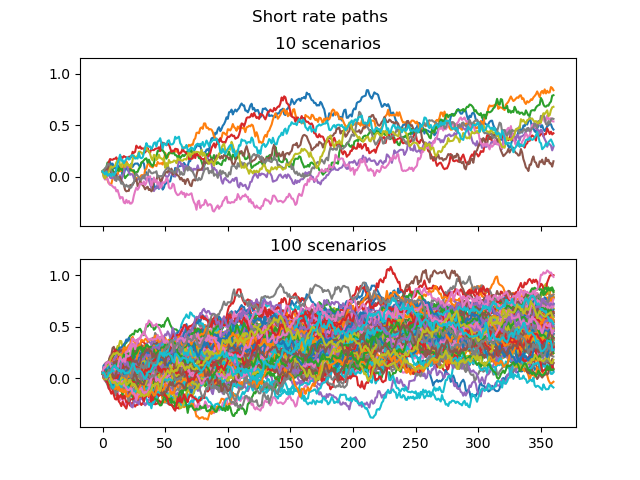# Short rate paths#

Short rate paths generated by the Monte Carlo simulation. The first 10 and 100 paths are plotted.```import modelx as mx
import matplotlib.pyplot as plt

paths = HW.short_rate_paths()

fig, axs = plt.subplots(2, 1, sharex=True, sharey=True)
fig.suptitle("Short rate paths")

for size, h in zip([10, 100], [0, 1]):
for i in range(size):
axs[h].plot(range(HW.step_size + 1), HW.short_rate_paths()[i])
axs[h].set_title(str(size) + ' scenarios')
```

Total running time of the script: ( 0 minutes 0.266 seconds)

Gallery generated by Sphinx-Gallery